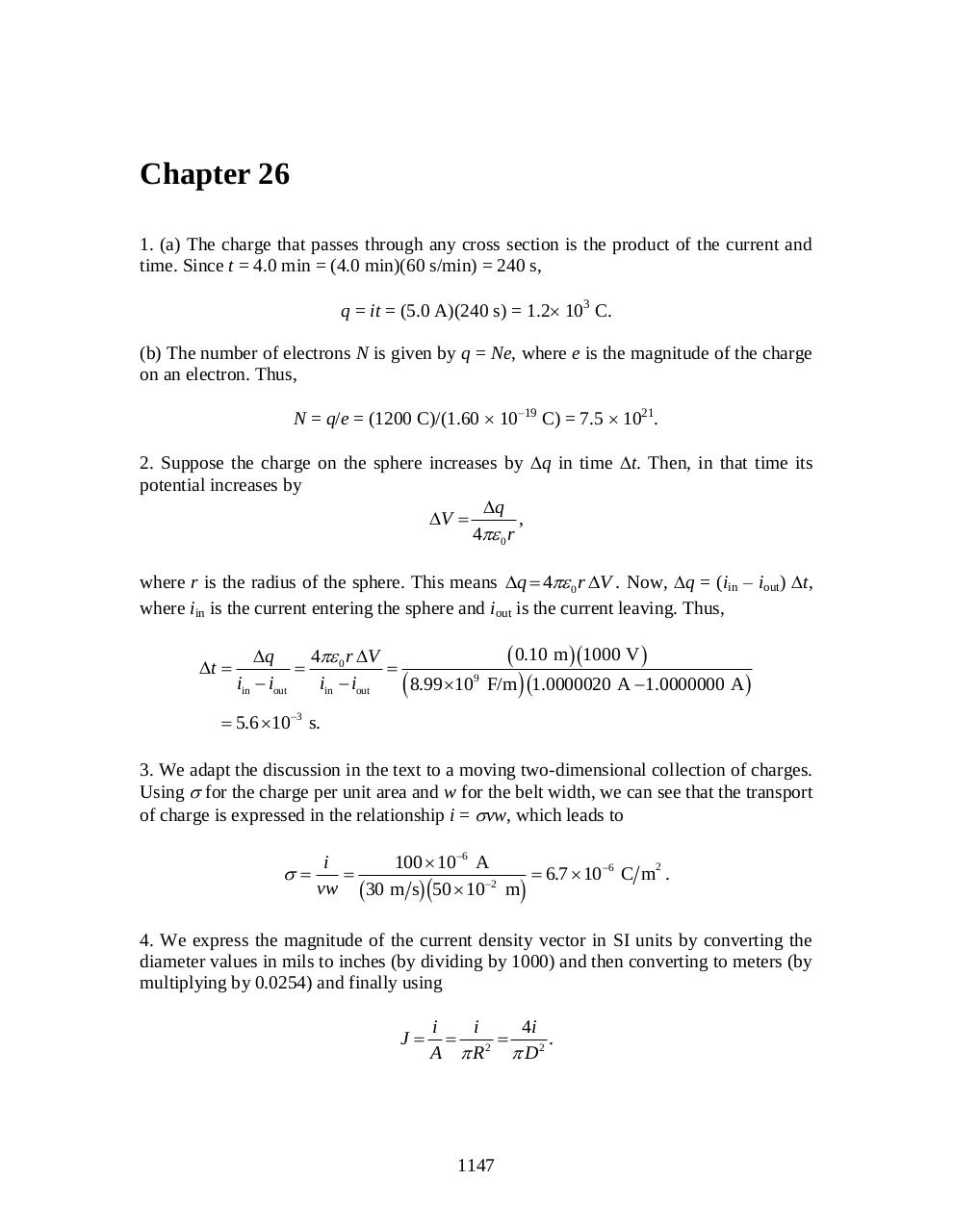# chapter 26.pdfPage 12326

#### Text preview

Chapter 26
1. (a) The charge that passes through any cross section is the product of the current and
time. Since t = 4.0 min = (4.0 min)(60 s/min) = 240 s,
q = it = (5.0 A)(240 s) = 1.2 103 C.
(b) The number of electrons N is given by q = Ne, where e is the magnitude of the charge
on an electron. Thus,
N = q/e = (1200 C)/(1.60  10–19 C) = 7.5  1021.
2. Suppose the charge on the sphere increases by q in time t. Then, in that time its
potential increases by
q
V 
,
4 0 r
where r is the radius of the sphere. This means q  4 0 r V . Now, q = (iin – iout) t,
where iin is the current entering the sphere and iout is the current leaving. Thus,

t 

 0.10 m 1000 V 
4 0 r V
q

9
iin  iout
iin  iout
8.99 10 F/m  1.0000020 A  1.0000000 A 

 5.6 103 s.
3. We adapt the discussion in the text to a moving two-dimensional collection of charges.
Using  for the charge per unit area and w for the belt width, we can see that the transport
of charge is expressed in the relationship i = vw, which leads to



i
100  106 A
2

 6.7  106 C m .
2
vw 30 m s 50  10 m

b

gc

h

4. We express the magnitude of the current density vector in SI units by converting the
diameter values in mils to inches (by dividing by 1000) and then converting to meters (by
multiplying by 0.0254) and finally using
J

i
i
4i

.
2
A  R  D2

1147